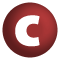# Success

#### class Success[+T](result: T, next: Reader[Elem]) extends ParseResult[T] with Product

The success case of ParseResult: contains the result and the remaining input.

result

The parser's output

next

The parser's remaining input

definition classes: Parsers

1. Product
2. Equals
3. ParseResult
4. AnyRef
5. Any

### Value Members

2. #### def canEqual(arg0: Any): Boolean

A method that should be called from every well-designed equals method that is open to be overridden in a subclass

A method that should be called from every well-designed equals method that is open to be overridden in a subclass. See Programming in Scala, Chapter 28 for discussion and design.

3. #### def equals(arg0: Any): Boolean

The equality method defined in `AnyRef`

The equality method defined in `AnyRef`.

5. #### def get: T

Returns the embedded result

Returns the embedded result

7. #### def hashCode(): Int

Returns a hash code value for the object

Returns a hash code value for the object.

The default hashing algorithm is platform dependent.

Note that it is allowed for two objects to have identical hash codes (`o1.hashCode.equals(o2.hashCode)`) yet not be equal (`o1.equals(o2)` returns `false`). A degenerate implementation could always return `0`. However, it is required that if two objects are equal (`o1.equals(o2)` returns `true`) that they have identical hash codes (`o1.hashCode.equals(o2.hashCode)`). Therefore, when overriding this method, be sure to verify that the behavior is consistent with the `equals` method.

9. #### def map[U](f: (T) ⇒ U): Success[U]

Functional composition of ParseResults

Functional composition of ParseResults

10. #### def mapPartial[U](f: PartialFunction[T, U], error: (T) ⇒ String): ParseResult[U]

Partial functional composition of ParseResults

Partial functional composition of ParseResults

error

a function that takes the same argument as ```f' and produces an error message to explain why ```f' wasn't applicable (it is called when this is the case)

returns

if `f' f is defined at the result in this `ParseResult', `f' applied to the result of this `ParseResult', packaged up as a new ```ParseResult'. If ```f' is not defined, `Failure'.`

12. #### def productArity: Int

return k for a product `A(x_1,`

``` return k for a product A(x_1,...,x_k) ```
``` ```
13. ``` def productElement(arg0: Int): Any for a product A(x_1, for a product A(x_1,...,x_k), returns x_(n+1) for 0 <= n < k ```
14. ``` def productElements: Iterator[Any] ```
15. ``` def productIterator: Iterator[Any] An iterator that returns all fields of this product An iterator that returns all fields of this product definition classes: Product ```
16. ``` def productPrefix: String By default the empty string By default the empty string. Implementations may override this method in order to prepend a string prefix to the result of the toString methods. ```
17. ``` val result: T ```
18. ``` val successful: Boolean ```
19. ``` def toString(): String The toString method of a Success @return a string representation of the object The toString method of a Success @return a string representation of the object. */ ```
``` ```
``` Instance constructors new Success(result: T, next: Reader[Elem]) new Success() ```
``` ```